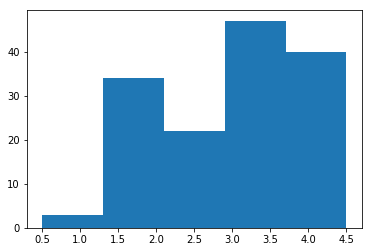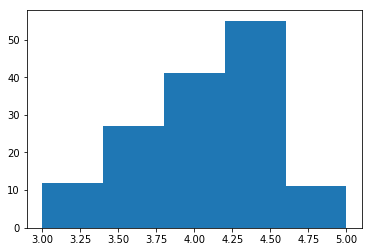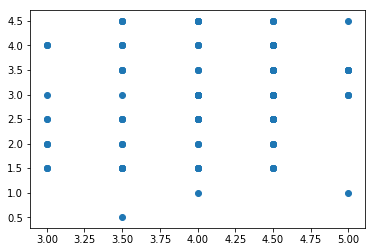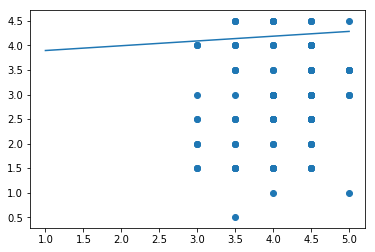In this project, we willl analyze various movie review websites using "fandango_score_comparison.csv" We will use descriptive statistics to draw comparisons between fandango and other review websites. In addition, we'll also use linear regression to determine fandango review scores based on other review scores.

In :
import pandas as pd
import matplotlib.pyplot as plt
import numpy as np


Out:
FILM RottenTomatoes RottenTomatoes_User Metacritic Metacritic_User IMDB Fandango_Stars Fandango_Ratingvalue RT_norm RT_user_norm ... IMDB_norm RT_norm_round RT_user_norm_round Metacritic_norm_round Metacritic_user_norm_round IMDB_norm_round Metacritic_user_vote_count IMDB_user_vote_count Fandango_votes Fandango_Difference
0 Avengers: Age of Ultron (2015) 74 86 66 7.1 7.8 5.0 4.5 3.70 4.3 ... 3.90 3.5 4.5 3.5 3.5 4.0 1330 271107 14846 0.5
1 Cinderella (2015) 85 80 67 7.5 7.1 5.0 4.5 4.25 4.0 ... 3.55 4.5 4.0 3.5 4.0 3.5 249 65709 12640 0.5
2 Ant-Man (2015) 80 90 64 8.1 7.8 5.0 4.5 4.00 4.5 ... 3.90 4.0 4.5 3.0 4.0 4.0 627 103660 12055 0.5
3 Do You Believe? (2015) 18 84 22 4.7 5.4 5.0 4.5 0.90 4.2 ... 2.70 1.0 4.0 1.0 2.5 2.5 31 3136 1793 0.5
4 Hot Tub Time Machine 2 (2015) 14 28 29 3.4 5.1 3.5 3.0 0.70 1.4 ... 2.55 0.5 1.5 1.5 1.5 2.5 88 19560 1021 0.5

5 rows × 22 columns

First, we'll use a histogram to see the distribution of ratings for "Fandango_Stars" and "Metacritic_norm_round".

In :
mc = movies['Metacritic_norm_round']
fd = movies['Fandango_Stars']

plt.hist(mc, 5)
plt.show()

plt.hist(fd, 5)
plt.show()It looks like fandango seems to have higher overalll ratings than metacritic, but just looking at histograms isn't enough to prove that. We can calclate the mean, median, and standard deviation of the two websites using numpy functions.

In :
mean_fd = fd.mean()
mean_mc = mc.mean()
median_fd = fd.median()
median_mc = mc.median()
std_fd = fd.std()
std_mc = mc.std()

print("means", mean_fd, mean_mc)
print("medians",median_fd, median_mc)
print("std_devs",std_fd, std_mc)

means 4.08904109589 2.97260273973
medians 4.0 3.0
std_devs 0.540385977979 0.990960561374


Couple of things to note here:

• Fandango rating methods are hidden, where as metacritic takes a weighted average of all the published critic scores.

• The mean and the median for fandango is way higher, they also got a low std deviation. I'd imagine their scores are influenced by studios and have inflated scores to get people on the website to watch the movies.

• The standard deviation for fandango is also lower because most of their ratings are clustered on the high side.

• Metacritic on the other hand has a median of 3.0 and an average of 3 which is basically what you would expect from a normal distribution.

Let's make a scatter plot between fandango and metacritic to see if we can draw any correlations.

In :
plt.scatter(fd, mc)
plt.show()In :
movies['fm_diff'] = fd - mc
movies['fm_diff'] = np.absolute(movies['fm_diff'])
dif_sort = movies['fm_diff'].sort_values(ascending=False)


Out:
FILM RottenTomatoes RottenTomatoes_User Metacritic Metacritic_User IMDB Fandango_Stars Fandango_Ratingvalue RT_norm RT_user_norm ... RT_norm_round RT_user_norm_round Metacritic_norm_round Metacritic_user_norm_round IMDB_norm_round Metacritic_user_vote_count IMDB_user_vote_count Fandango_votes Fandango_Difference fm_diff
3 Do You Believe? (2015) 18 84 22 4.7 5.4 5.0 4.5 0.90 4.20 ... 1.0 4.0 1.0 2.5 2.5 31 3136 1793 0.5 4.0
85 Little Boy (2015) 20 81 30 5.9 7.4 4.5 4.3 1.00 4.05 ... 1.0 4.0 1.5 3.0 3.5 38 5927 811 0.2 3.0
47 Annie (2014) 27 61 33 4.8 5.2 4.5 4.2 1.35 3.05 ... 1.5 3.0 1.5 2.5 2.5 108 19222 6835 0.3 3.0
19 Pixels (2015) 17 54 27 5.3 5.6 4.5 4.1 0.85 2.70 ... 1.0 2.5 1.5 2.5 3.0 246 19521 3886 0.4 3.0
134 The Longest Ride (2015) 31 73 33 4.8 7.2 4.5 4.5 1.55 3.65 ... 1.5 3.5 1.5 2.5 3.5 49 25214 2603 0.0 3.0

5 rows × 23 columns

It looks like the difference can get as high as 4.0 or 3.0. We should try to calculate the correlation between the two websites. We can do this by simply using the .pearsonr() function from scipy.

In :
import scipy.stats as sci

r, pearsonr = sci.pearsonr(mc, fd)
print(r)
print(pearsonr)

0.178449190739
0.0311615162285


If both movie review sites uses the similar methods for rating their movies, we should see a strong correlation. A low correlation tells us that these two websites have very different review methods.

Doing a linear regression wouldn't be very accurate with a low correlation. However, let's do it for the sake of practice anyway.

In :
m, b, r, p, stderr = sci.linregress(mc, fd)

#Fit into a line, y = mx+b where x is 3.
pred_3 = m*3 + b
pred_3

Out:
4.0917071528212041
In :
pred_1 = m*1 + b
print(pred_1)
pred_5 = m*5 + b
print(pred_5)

3.89708499687
4.28632930877


We can make predictions of what the fandango score is based on the metacritic score by doing a linear regression. However it is important to keep in mind, if the correlation is low, the model might not be very accurate.

In :
x_pred = [1.0, 5.0]
y_pred = [3.89708499687, 4.28632930877]

plt.scatter(fd, mc)
plt.plot(x_pred, y_pred)

plt.show()#### Learning Summary¶

Concepts explored: pandas, descriptive statistics, numpy, matplotlib, scipy, correlations

Functions and methods used: .sort_values(), sci.linregress(), .hist(), .absolute(), .mean(), .median(), .absolute()

The files used for this project can be found in my GitHub repository.